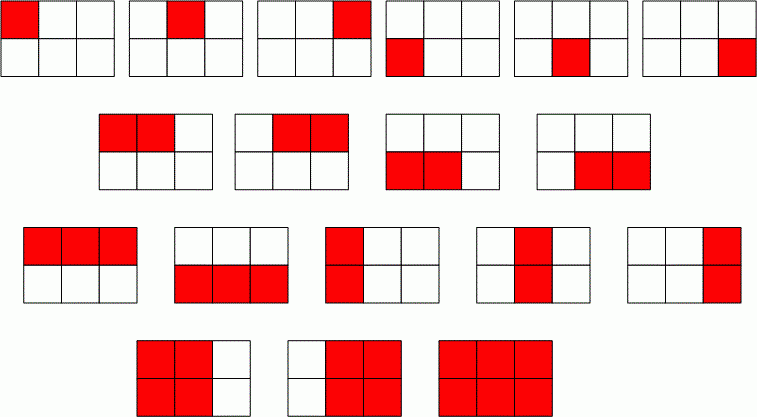Competitions

# Number of rectangles

The grid consists of n * m unit squares. How many different rectangles can be drawn on it?

The sides of the rectangles should be parallel to the grid lines. The rectangle must cover an integer number of squares. Two rectangles of the same size are considered different if they are located in different places on the grid.

#### Input

Two positive integers n and m (n, m105).

#### Output

Print the number of different rectangles that can be drawn on the grid.Time limit 1 second
Memory limit 128 MiB
Input example #1
2 3

Output example #1
18

Author Michael Medvediev